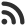Feed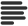Articles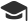Tutorials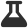Lab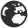CompaniesLeaderboard
 DG Student at BIT Mesra Aug. 24, 2020, 1:31 p.m. ⋅ 735 views

# LTI placement Logical Reasoning Questions with answers

1. If P ≤ Q < R = S ≤ T > U ≥ V, which of the following is definitely false?

A. S>U
B. P>S
C. R>U
D. R>V

Ans. B

2. P + Q means P is the brother of Q; P – Q means P is the mother of Q and P * Q means P is the sister of Q.
Which of the following means M is the maternal uncle of R ?

A. M + K + R
B. M – R + K
C. M + K – R
D. M + K * R

Ans. C

3. In a queue, Amrita is 10th from the front while Mukul is 25th from behind and Mamta is just in the middle of the two. If there be 50 persons in the queue. What position does Mamta occupy from the front ?

A. 20 th
B. 19 th
C. 18 th
D. 17th

Ans. C

4. A man walks 40 meters towards north. Then turning to his right, he walks 50 meter. Then turning to his left, he walks 30 meters. Again he turns to his left and walks 40 meters. How far is he from initial position?

A. 40√2
B. 50√2
C. 60√2
D. 50√3

Ans. B

5. A person starts walking from his home in west direction and after walking 20 meter he took a left turn and walk 30 meters. Now he took a right turn and walks 10 meter to reach the bus stand. Find the distance between home and stand.

A. 20√2
B. 30√2
C. 40√2
D. 50√2

Ans. B

Find the next number in the series :

6. 9,12,4,8,12,15,5,9,3,6,2,6,6,6

A. 4
B. 5
C. 6
D. 3

Ans. D

7. 1,3,5,9,17,33

A. 35
B. 37
C. 55
D. 65

Ans. D

8. 8,16,3,27,4,8,7,19,2,0,7,

A. 7
B. 9
C. 11
D. 13

Ans. B

9. 5,6,6.5,6.5,8,7,9.5

A. 5.5
B. 6.5
C. 7.5
D. 11.5

Ans. C

10. 9,3,24,4,9,3,26,6,9,3,30

A. 5
B. 10
C. 12
D. 11

Ans. B

11. 30.5,31,30,0,67.2,67,70,100,183.9

A. 164
B. 174
C. 184
D. None of these

Ans. C

12. 2,3,6,13,28

A. 59
B. 56
C. 55
D. 54

Ans. A

13. 2,9,23,79,415

A. 1250
B. 1245
C. 2500
D. 3103

Ans. D

14. 1,2,3,6,11,20,37,68

A. 120
B. 125
C. 250
D. 310

Ans. B

15. 1.8,3,0.6,2.5,5.0,0.5,3.4,2,1.7

A. 5.5
B. 6.5
C. 4.5
D. None of these

Ans. C

Events

Nov. 28, 2018, 5:30 p.m.

Python from zero to hero

place Delhi

Aug. 13, 2018, 5:30 p.m.

Python from zero to hero

place Bangalore ( HackersFriend office BTM Layout)